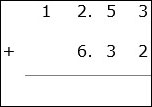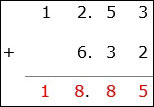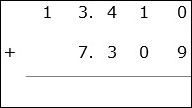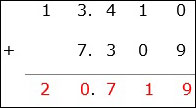The decimals are aligned so that the digits of same place values are above and below each other. Such decimals are aligned decimals. It is necessary that the decimals are aligned before addition. Addition of aligned decimals is similar to addition of whole numbers.

• Aligning the decimal numbers so that digits of same place values are lined up vertically one above the other

• Adding zeros to the right of the decimal numbers where ever necessary.

So, at first the decimals are aligned so that the digits of same place values are above and below each other. Then zeros are added to the right of decimal numbers as required. We begin with the rightmost column and start adding the digits. Then we keep moving to the left till all columns are added.

### Solution

Step 1:The decimals are aligned as shown.

Step 2:

We start with the right most column. Add 3 + 2 = 5; then moving to left column 5 + 3 = 8; again 2 + 6 = 8; next 1 + 0 = 1.

Step 3:

So the sum of the decimals is 18.85### Solution

Step 1:The decimals are aligned as shown. A zero is added to the right of 13.41 as the maximum number of decimal places is 3.

Step 2:

We start with the right most column. Add 0 + 9 = 9; then moving left 1 + 0 = 1; again 4 + 3 = 7; next 3 + 7 = 10 we bring down 0; carry 1 to next column. 1 + 1 = 2;Step 3:

So the sum of the decimals is 20.719